Time：2019-12-2

# Recommendation system based on coupling network

Author: Chen Dongrui

## 1. Basic knowledge of complex network

When we pick up our mobile phones to call our family, friends or colleagues, we unconsciously participate in the process of social network formation; when we board the high-speed rail or the plane, we can enjoy the convenience brought by the traffic network; even when we lie in bed and do nothing, the neurons in the brain will form a huge complex network to transmit to each other Signals that help us think or act. Complex network is a theoretical tool that abstracts all kinds of large-scale complex systems in the real world into networks for research. A large number of complex systems in nature can be described by various kinds of networks.### 1.1 representation of network

A typical network is composed of many connecting edges between nodes, in which nodes are used to represent different individuals in the real system, while edges are used to represent the relationship between nodes. Usually, if there is a certain relationship between two nodes, one edge is connected, otherwise there is no connection, and the two nodes connected at the edge are regarded as adjacent in the network. In order to facilitate the calculation, we usually use adjacency matrix to represent the network. According to the different types of network edges, the network can be divided into undirected network, directed network and weighted network. The corresponding adjacency matrix is shown as follows:### 1.2 statistical characteristics of network

• degree: the number of sides directly connected to the node; in a directed network, it can be divided into outgoing and incoming degrees.
• convergence factor: the possibility that the neighbors of a node are neighbors to each other, which measures the degree of network clustering

$$C  I = \ frac {the number of edges actually connected between neighbors of this node} {the maximum possible number of connected edges K  I (K  i-1) / 2}$$

We can get the average aggregation coefficient by calculating the arithmetic mean of the aggregation coefficient, and then measure the aggregation degree of the whole network.

• Shortest path: the shortest connecting path between two nodes
• Betweenness: the medium number includes the node medium number and the edge medium number.

• Node medium refers to the proportion of the number of shortest paths passing through the node in the network
• The number of edges refers to the proportion of the number of shortest paths passing through the edge in the network
• The medium number reflects the function and influence of the corresponding node or edge in the whole network

### 1.3 common complex network models

The commonly used network models include regular network, random network, small world network and scale-free network.

• Regular network

Regular network is the simplest network model. In this type of network, the connection between any two nodes follows the established rules, usually the number of neighbors of each node is the same.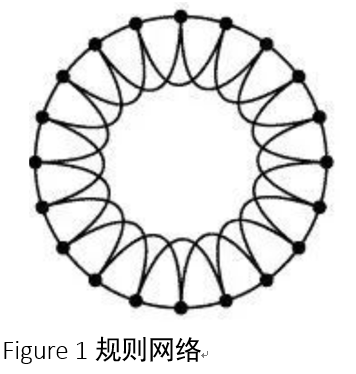• Stochastic network

It is completely random whether there are connected edges between nodes.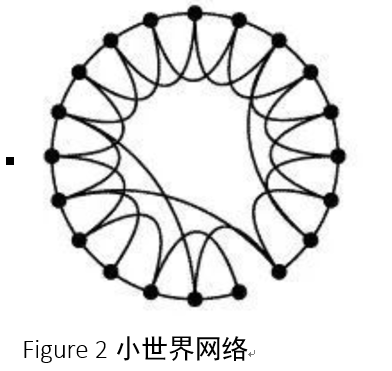• Small world network

The small world network model was proposed by Watts and Strogatz in the 1998 paper collective dynamics of small world networks published in nature. They found that the clustering of regular network is high, but the average distance of network is also large, while the average distance of random network is short, and its clustering is also low. The real world network is neither completely regular nor completely random, but between the two, so some scholars introduced the small world network model.• Scale free network

Scale-free network is a network in which most nodes (small nodes) only connect with a few nodes, while a few nodes connect with a lot of nodes (large nodes).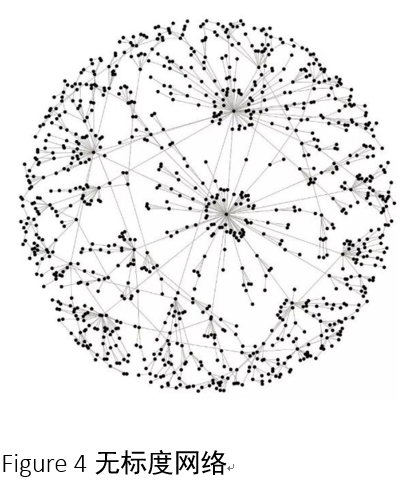## Background of recommendation system based on complex network

Link prediction refers to how to predict the possibility of connection between two nodes in the network that have not yet generated a connection edge through known network structure and other information. The prediction of existing but not yet discovered connections is actually a data mining process, while the prediction of possible future connections is related to the evolution of the network. Link prediction can be applied to e-commerce websites. If the goods in the e-commerce website are regarded as one kind of node and the user as another kind of node, if user a purchases goods B, a and B will form a connecting edge. This edge only exists between different types of nodes and becomes a binary network, and the chain path prediction problem in the binary network is actually a kind of recommendation system.Now let’s briefly introduce an article of recommendation system based on complex network, information filtering via based random walk on coupled social
Network》。 In this paper, the user’s social network and the user commodity binary graph network are coupled, and the user’s social information and commodity preference information are integrated to recommend commodities to users.
Coupling social network (CSN) includes coupling nodes (users), which form leader follower relationship in social network layer and collection relationship in information network layer. The figure below is a simple schematic diagram of a coupled social network, with circles representing users and squares representing objects. The first half is the social network of five users. The $U4$refers to the connection of $U5$. The $U4$is the follower of $U5$. There is a certain degree of similarity between them. The second half is a binary network. There is a connection between the object $O5$and the user $U5$, indicating that the user $U5$has $O5$. If there is only the second half of the network, we can not recommend the product $O5$to the user $U4$. When we consider the similarity between $U4$and $U5$in the social network, we can recommend the object $O5$to the user $U4$. Next, let’s explain how this method works in the recommendation system.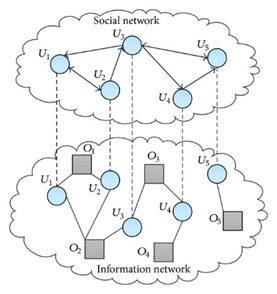## 3. Model introduction

For a recommendation system, we divide it into two parts: user set $u = \ {u  1, u  2,…, u  m \}$and object set $o = \ {o  1, O  2,…, O  n \}$, indicating that there are $M$users and $n$objects. The adjacency matrix $a {m * n}$is defined to represent the network,
$$a_{ialpha} = begin{cases} 1 & the user has collected the object o alpha\ 0 & user has no favorite object o alpha end{cases}$$
The adjacency matrix $B {m * n}$represents the user object bipartite graph,
$$b_{ij} = begin{cases} 1 & user (or object) I has collected object (or user) J\ 0 & others end{cases}$$

### 3.1 random walk on social network

• $p {I j} ‘$is the transition probability on social network, from user $u {I$to user $u {J$:

$$P_{ij}’ = begin{cases} frac{b_{ij}}{k_j^{out}} & if k_j^{out} neq 0 \ 0 & otherwise end{cases}tag{1}$$

• $s’I ‘(T)$indicates the probability that other users will arrive at user $u’i$at time t,

$$S_i’ (t+1) = begin{cases} sum_{j = 1}^{m} frac{b_{ij}}{k_j^{out}} & if k_j^{out} neq 0 \ 0 & otherwise end{cases}tag{2}$$

• Initial probability

• For target user $u_i$, $s_i ‘(0) = 1$
• For other users $u_j$, $s_j ‘(0) = 1$
#Travel on social networks
#Input user ID, probability prob, Lama in the paper, step of walk
user_id = '23298'
prob = 1
step = 3
# def social_network_walk(user_id, prob, step):
user_group = trust_df.groupby(trust_df)

#The first step
id_neighbors = list(user_group.get_group(user_id).values)
user_prob_dic = {}
for user_id in id_neighbors:
user_prob_dic[user_id] = prob/len(id_neighbors)
user_dict =  user_prob_dic.copy()
#After the walk
for _ in range(step-2):
for item in user_dict.items():
uesr_id = item
prob = item
id_neighbors = list(user_group.get_group(user_id).values)
for user_id in id_neighbors:
try:
user_prob_dic[user_id] += prob/len(id_neighbors)
except KeyError:
user_prob_dic[user_id] = prob/len(id_neighbors)
user_dict =  user_prob_dic.copy()
# return user_dict
user_dict

### 3.2 random walk on bipartite graph

• Transfer probability of users to goods:
$$P_{ialpha}’ = begin{cases} frac{a_{ialpha}}{k_i} & if k_i neq 0 \ 0 & otherwise end{cases}tag{3}$$
• Transfer probability of goods to users:
$$P_{alpha j}” = begin{cases} frac{a_{jalpha}}{k_{alpha}} & if k_{alpha} neq 0 \ 0 & otherwise end{cases}tag{4}$$
• Define $s {\ alpha} ” (T)$, and $s {J} ” (T)$, as the probability of commodity $\ alpha$, user $t$, in bipartite graph at the time:

$$S_i’’ (t+1) = begin{cases} sum_{alpha = 1}^{m} frac{a_{ialpha}}{k_{alpha}} S_{alpha}”(t)& if k_{alpha} neq 0 \ 0 & otherwise end{cases}tag{5}$$

$$S_{alpha}’’ (t+1) = begin{cases} sum_{j = 1}^{m} frac{a_{jalpha}}{k_j} S_j”(t) & if k_j neq 0 \ 0 & otherwise end{cases}tag{6}$$
When $t$is an odd number and $t \ geq3$, $s {\ alpha} “(T)$, indicates the probability that the user $u {\ alpha}” (the user with the initial value set to 1) will select the uncollected product$o {\ alpha} $. #Wandering on bipartite graph network #The first step is to travel from users to commodities # def bipartite_walk(user_id, perct): user_id = '23298' prob = 1 Object group = ratings DF. Group by (ratings DF ) classifies the objects based on the user's no basis. The same user's favorite objects User group = ratings DF. Group by (ratings DF ) classifies users based on objects, which users collect the same object based on objects objection_dict = {} user_dict = {} #Step 1 user to object User? Neighbors = object? Group. Get? Group (user? ID) . Values? User's neighbors are objects for objection_id in user_neighbors: print(objection_id) objection_dict[objection_id] = prob/len(user_neighbors) #Step 2 object to user # objection_lis = objection_dict.keys() for item in objection_dict.items(): objection_id = item prob = item objection_neighbors = user_group.get_group(objection_id).values for user_id in objection_neighbors: try: user_dict[user_id] += prob/len(objection_neighbors) except KeyError: user_dict[user_id] = prob/len(objection_neighbors) # # #Step 3: user to object for item in user_dict.items(): user_id = item prob = item user_neighbor = list(objection_group.get_group(user_id).values) for obj_id in user_neighbor: try: objection_dict[obj_id] += prob/len(user_neighbor) except KeyError: objection_dict[obj_id] = prob/len(user_neighbor) objection_dict ### 3.3 biased random walks on coupled social networks In the coupling network, from users in social networks and commodities in the bipartite graph network to users in the bipartite graph network$$S_{alpha}(t+1) = begin{cases} sum_{j=1}^{m} frac{a_{jalpha}}{k_j}S_j(t) & if k_j neq 0 \ 0 & otherwise end{cases}tag{8}$$ Initial probability: •$u i $,$s I ” (0) = 1 for target user$• For other users$u $and products$\ alpha $,$s_j (\ alpha) = 0 $,$s {\ alpha} = 0$When$t $is an odd number and$t \ GEQ 3 $,$s {\ alpha} “(T) $represents the probability that the user$u_i $, selects the uncollected product$o {\ alpha} $(set the initial value of the target user as 1 unit resource, when$t = 1 $, the resource will swim from the target user to the adjacent object; when$t = 2 $, the resource will swim from the object to the user again). #Coupling network walk, user 23298 random walk in coupling network, step size is three, transfer probability is 0.7 user_id = '23298' prob_social = 0.7 step = 3 prob_bi = 1-prob_social social_user_group = trust_df.groupby(trust_df) #Classify the objects based on the user's unsubstantiation. The same user's favorite objects bi_objection_group = ratings_df.groupby(ratings_df) #Based on the object, classify users, based on the object, which users collect the same object bi_user_group = ratings_df.groupby(ratings_df) objection_dict = {} user_dict = {} #Step 1 user to object User? Neighbors = Bi? Object? Group. Get? Group (user? ID) . Values? User's neighbors are objects for objection_id in user_neighbors: objection_dict[objection_id] = prob_social/len(user_neighbors) for _ in range(int((step-1)/2)) : #Step 2: object to user for item in objection_dict.items(): objection_id = item prob = item objection_neighbors = bi_user_group.get_group(objection_id).values for user_id in objection_neighbors: try: user_dict[user_id] += prob/len(objection_neighbors) except KeyError: user_dict[user_id] = prob/len(objection_neighbors) user_neighbors = list(social_user_group.get_group(user_id).values) for user_id in user_neighbors: try: user_dict[user_id] += prob_social/len(user_neighbors) except KeyError: user_dict[user_id] = prob_social/len(user_neighbors) #Step 3: user to object for item in user_dict.items(): user_id = item prob = item user_neighbor = list(objection_group.get_group(user_id).values) for obj_id in user_neighbor: try: objection_dict[obj_id] += prob/len(user_neighbor) except KeyError: objection_dict[obj_id] = prob/len(user_neighbor) objection_dict Evaluation index: •$precision $, the proportion of the product selected by the user in the recommendation list.$precision ^i= N_{rs}^i/Ln {RS} ^ I $: the number of items recommended for user$u {I $: in the test set,$l $: the length of the recommendation list. •$recall $, the proportion of recommended items in the user’s favorite list.$recall^i = N_{rs}^i/N_p^i$，$n ^ p ^ I $: number of items in the test set that user$u ^ I $•$F-measure = \frac{2*Precision \times Recall}{Precision+Recall}$•$HD $Hamming distance, a measure of the diversity of user recommendation lists.$HD {I j} = 1 – Q {ij} (L) / L $,$Q {ij} $, is the quantity of the same product in the recommendation list of user$I $and user$J $. •$ranking score (R) $: measures users’ satisfaction with the recommendation list,$R {I \ alpha} = l {I \ alpha} / N {I} $.$l {I \ alpha} $, is the user’s location in the user’s$I $recommendations list for items$\ alpha $.$n_i $is the length of the recommended list. ## 4. Result analysis ### 4.1 data description The two public data sets of epicions and FriendFeed contain a social relationship data set and a scoring data set. The following table describes the network corresponding to the dataset. Taking epinations as an example, a part of data is randomly selected for analysis. The sampling data scale is 4066 users, 7649 objects, 154122 user object connections (collections), and 217017 social network connections. The network density is 5×10-3. Table 1: Properties of the tested data sets### 4.2 effect of parameters$\ lambda $and$t $, on experimental results$\ lambda $is the allocation proportion of resources in social networks and bipartite graph networks, and$t $is the walk step length. The following figure is measured by sorting points. The colder the value color is, the better the effect is. It can be seen in the figure that when $$t = 3$$, the better the prediction effect is. When$\ lambda = 0 $, the prediction effect only depends on the bipartite graph network. With the increase of$\ lambda $, the information of social network is gradually considered. Figure1:Ranking score values on Epinions and Friendfeed data sets (color online).(a)Epinions （b）Friendfeed ### 4.3 prediction effect The following table shows the effect comparison of the model with MD (mass diffusion) model and UCF (user based CF) model when the predicted length is$l = 20 \$.

Table2 Algorithmic performance for Epinions data set with recommendation list L =20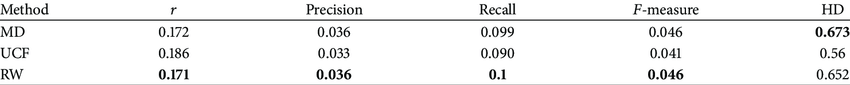Table3 Algorithmic performance for Friendfeed data set with recommendation list L =20This project has been implemented on Mo platform. Remember to fork:https://momodel.cn/explore/5d…

## [](https://www.yuque.com/docs/sh…